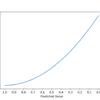# Archive | Python Machine Learning## What Is Semi-Supervised Learning

Semi-supervised learning is a learning problem that involves a small number of labeled examples and a large number of unlabeled examples. Learning problems of this type are challenging as neither supervised nor unsupervised learning algorithms are able to make effective use of the mixtures of labeled and untellable data. As such, specialized semis-supervised learning algorithms […]## Sensitivity Analysis of Dataset Size vs. Model Performance

Machine learning model performance often improves with dataset size for predictive modeling. This depends on the specific datasets and on the choice of model, although it often means that using more data can result in better performance and that discoveries made using smaller datasets to estimate model performance often scale to using larger datasets. The […]## Regression Metrics for Machine Learning

Regression refers to predictive modeling problems that involve predicting a numeric value. It is different from classification that involves predicting a class label. Unlike classification, you cannot use classification accuracy to evaluate the predictions made by a regression model. Instead, you must use error metrics specifically designed for evaluating predictions made on regression problems. In […]## A Gentle Introduction to Machine Learning Modeling Pipelines

Applied machine learning is typically focused on finding a single model that performs well or best on a given dataset. Effective use of the model will require appropriate preparation of the input data and hyperparameter tuning of the model. Collectively, the linear sequence of steps required to prepare the data, tune the model, and transform […]## Semi-Supervised Learning With Label Spreading

Semi-supervised learning refers to algorithms that attempt to make use of both labeled and unlabeled training data. Semi-supervised learning algorithms are unlike supervised learning algorithms that are only able to learn from labeled training data. A popular approach to semi-supervised learning is to create a graph that connects examples in the training dataset and propagates […]## Multinomial Logistic Regression With Python

Multinomial logistic regression is an extension of logistic regression that adds native support for multi-class classification problems. Logistic regression, by default, is limited to two-class classification problems. Some extensions like one-vs-rest can allow logistic regression to be used for multi-class classification problems, although they require that the classification problem first be transformed into multiple binary […]## Semi-Supervised Learning With Label Propagation

Semi-supervised learning refers to algorithms that attempt to make use of both labeled and unlabeled training data. Semi-supervised learning algorithms are unlike supervised learning algorithms that are only able to learn from labeled training data. A popular approach to semi-supervised learning is to create a graph that connects examples in the training dataset and propagate […]## Perceptron Algorithm for Classification in Python

The Perceptron is a linear machine learning algorithm for binary classification tasks. It may be considered one of the first and one of the simplest types of artificial neural networks. It is definitely not “deep” learning but is an important building block. Like logistic regression, it can quickly learn a linear separation in feature space […]## A Gentle Introduction to PyCaret for Machine Learning

PyCaret is a Python open source machine learning library designed to make performing standard tasks in a machine learning project easy. It is a Python version of the Caret machine learning package in R, popular because it allows models to be evaluated, compared, and tuned on a given dataset with just a few lines of […]## How to Identify Overfitting Machine Learning Models in Scikit-Learn

Overfitting is a common explanation for the poor performance of a predictive model. An analysis of learning dynamics can help to identify whether a model has overfit the training dataset and may suggest an alternate configuration to use that could result in better predictive performance. Performing an analysis of learning dynamics is straightforward for algorithms […]